1.   It's hard to tell the truth without statistics!

Statistical analysis is a crucial component of data analytics useful in interpreting the behavior of data and uncovering the patterns and trends underlying it. It assists in describing the nature of the data to be analyzed and exploring the relationships among the data attributes. Furthermore, the tests performed for statistical analysis proves (or disproves) the validity of the statistical model. There is a wide range of statistical tests which can be performed and the choice of which relies upon the structure and nature of the data and the variable type. This post focuses on Independent samples T-test and inferring data using this test using Smarten Augmented Analytics.

2.   A hypothesis is an idea which can be tested!

A Hypothesis test is a statistical test that is used to determine whether there is enough evidence in a sample of data to infer that a certain condition is true for the entire population. The foremost step in any hypothesis test is to define the Null hypothesis (H₀) and Alternate hypothesis (H₁) and then perform the respective test which will either reject the defined Null hypothesis and accept the alternate hypothesis, or accept the null hypothesis and reject the alternate hypothesis. Null hypothesis (H₀) is an argument we believe to be true even before we collect any data.  Alternate hypothesis (H₁) is an argument we want to prove to be true.

3.   The best way to understand is by an example!

Let’s consider that we have a car valuation dataset with a good deal of attributes influencing car valuation. Now we want to conduct a hypothesis test to analyze the variability in car prices based upon say features like availability of Air Condition facilities, the transmission type of the car (i.e., manual or automatic), past accident history, insurance claims or the car owner type (first owner or second owner). Let’s consider taking into account car price and car transmission type attributes for instance. Now this relationship can be evaluated using Hypothesis testing by defining our hypothesis as follows:Car Valuation Dataset - Smarten

Null hypothesis (H₀): These is not much difference in car price based upon car transmission type.

Alternate hypothesis (H₁): There is significant difference in car price based upon car transmission type.

So, on performing the statistical tests on our car valuation data, we get to either accept or reject the null hypothesis. In order to quantify this identification for our hypothesis, we need to determine the significance level (?) which is a probability of rejecting our null hypothesis, provided it's actually true. Any statistical test we perform to make conclusions for our hypothesis, calculates a p-value, which describes whether the outcome of the statistical test is statistically significant.

Low p-value: Your sample results are not consistent with a null hypothesis.

High p-value: Your sample results are consistent with a true null hypothesis.

This implies that, smaller the p-value, the sample is inconsistent with the null hypothesis and one can reject the null hypothesis.

4.   Independent sample T-test:

Also known as unpaired samples T-test, this hypothesis test is applicable in order to create comparative analysis of the means of two samples to check if both samples differ significantly or not. Independent samples T-Test can be used when we have one categorical independent variable with 2 different categories(e.g., car transmission type be the independent variable with Automatic and Manual being categories) and one quantitative dependent variable (e.g., car price).

5.   Performing Independent Samples T-test using Smarten:

Having an understanding about the Independent samples T-test and knowing its terminologies broadly, let’s dive swiftly to performing this test straightforwardly using Smarten Augmented Analytics!

5.1   Create a New Smarten Insight

Go to New -> SmartenInsight Menu in the dropdown provided in the top right corner of the Smarten Dashboard.Creating a new Smarten Insight

5.2     Select data to perform Independent Samples T-test upon and click NEXTSearch and Select dataset to Perform Independent Samples T-test

5.3     Perform data pre-processing steps i.e., sampling and filtering if required and click NEXTData Pre-Processing using Smarten

5.4     Handle Outliers and Missing Values if required and click NEXT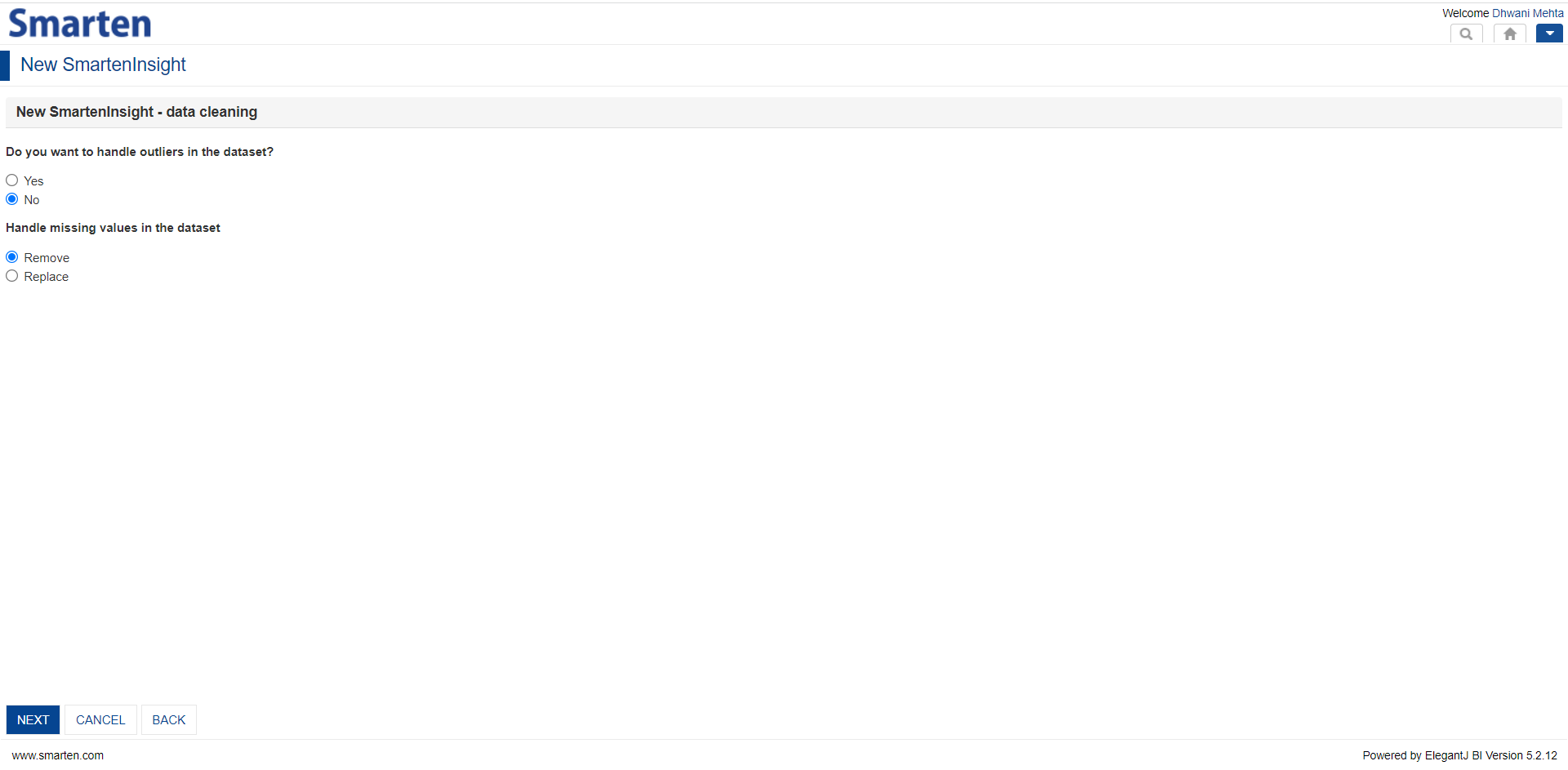Handling Outliers and Missing Values using Smarten

5.5    What do you want to do?

After performing all the data processing and handling steps, Smarten asks users to perform their respective algorithmic technique to proceed with. Let’s select the Hypothesis testing.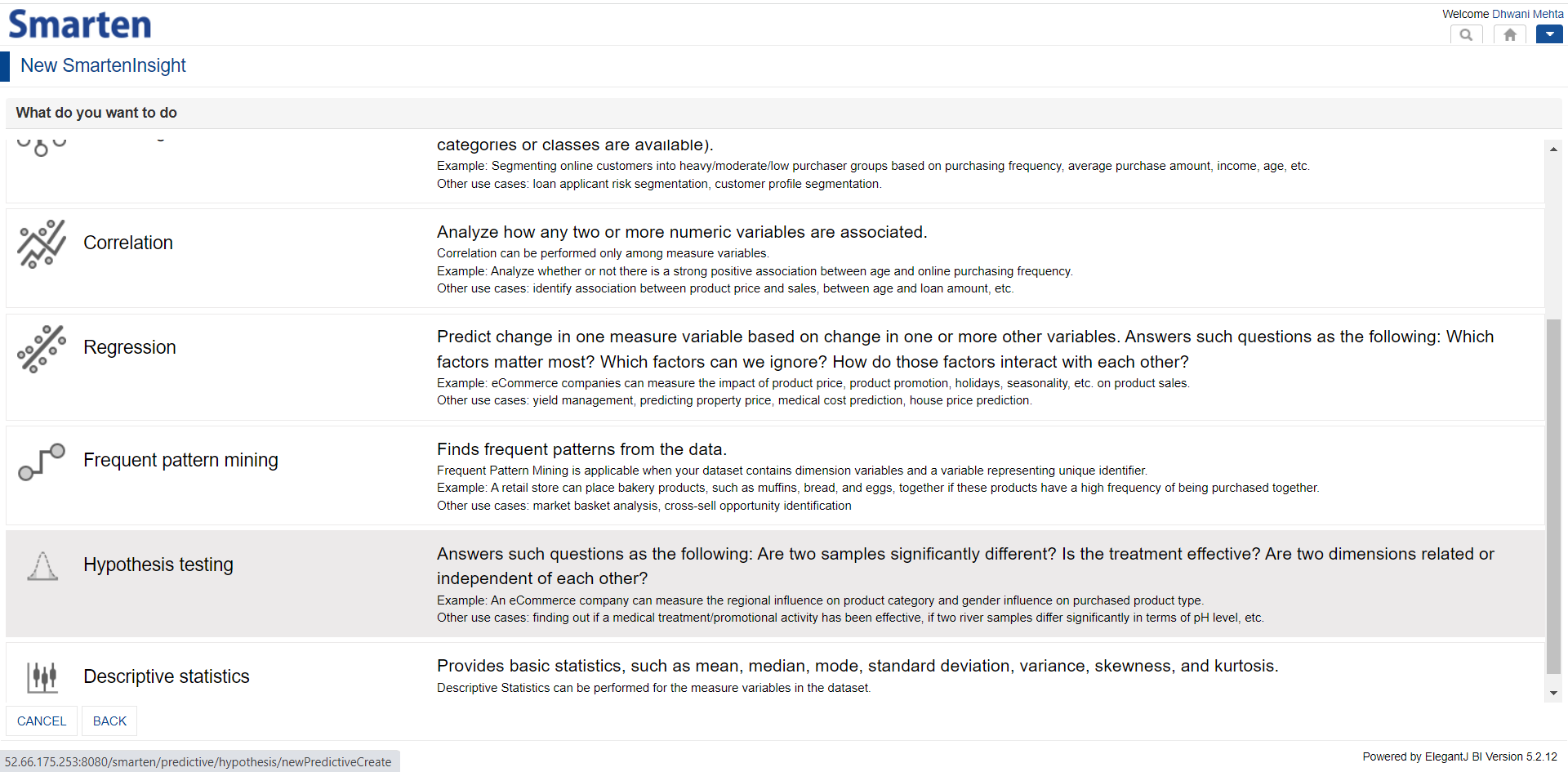Ask Smarten to Perform Hypothesis testing

5.6    Smarten knows about our objective and automates performance of corresponding best fit hypothesis test

As described earlier, Independent samples T-test is used to analyze if there is statistically significant difference among 2 samples and this test can be used when we have one categorical independent variable with 2 different categories (e.g., car transmission type being the independent categorical variables having categories as Manual and Automatic) and one quantitative dependent variable (e.g., car price). Let’s select our variables accordingly and proceed by clicking NEXT!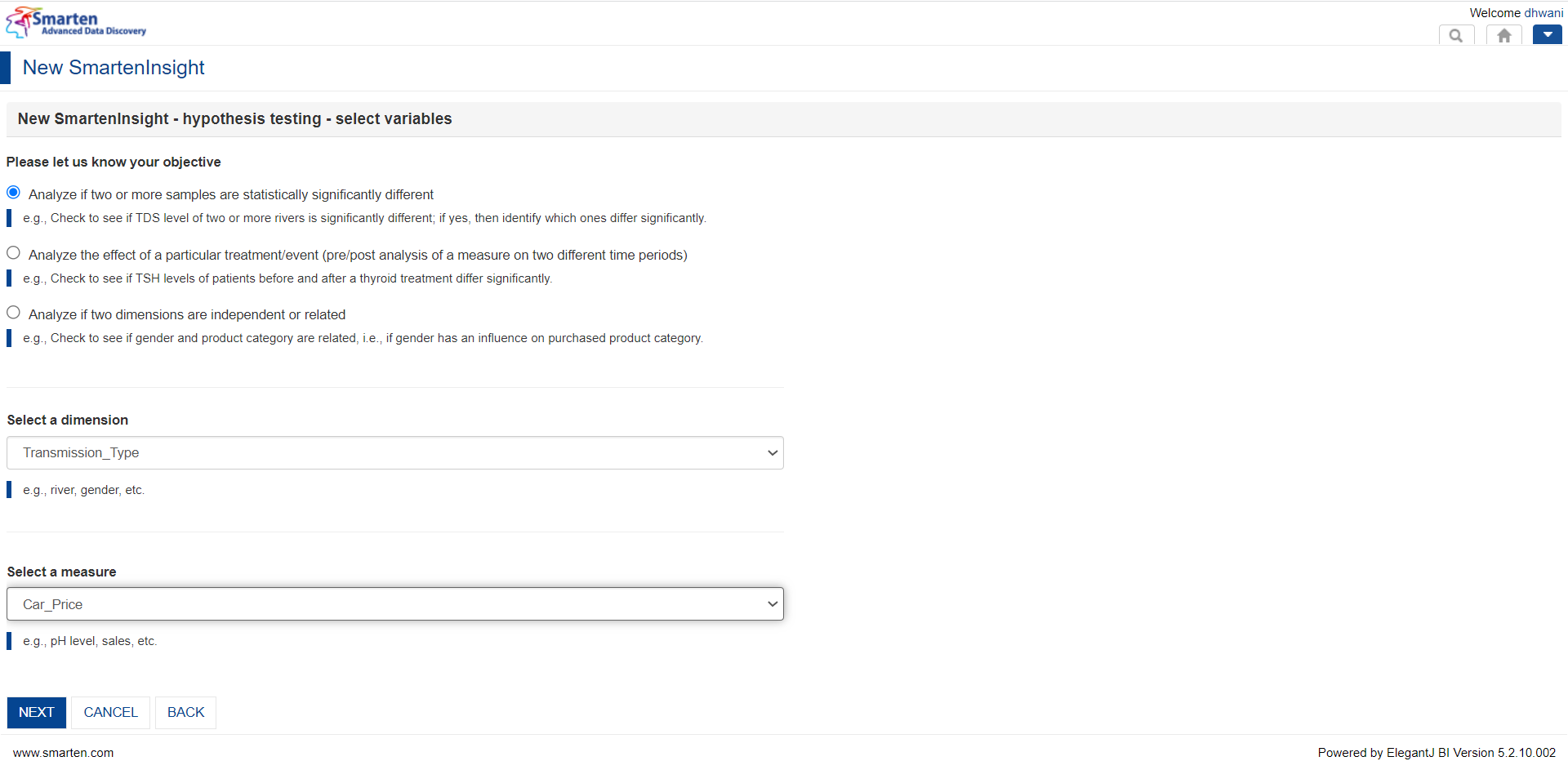Variable selection for Hypothesis testing

6.   And here we go! Independent samples T-test performed using Smarten Assisted Predictive Modeling!

Independent samples T-test test performed and the corresponding visualization allows users to get an easy insight upon how the group means vary.Independent samples T-test using Smarten

The above screen evidently explains that cars with automatic transmission type are costlier as compared to those with manual transmission type. Also, the P value being 0.0002 which is lesser than the default set significance threshold (i.e., 0.05) further validates the Alternate hypothesis (H₁).

7.   Interpretation of Independent samples T-test

In order to understand the insights from the hypothesis performed, navigate to the Interpretation tab to understand the variability among the chosen variables in simple language.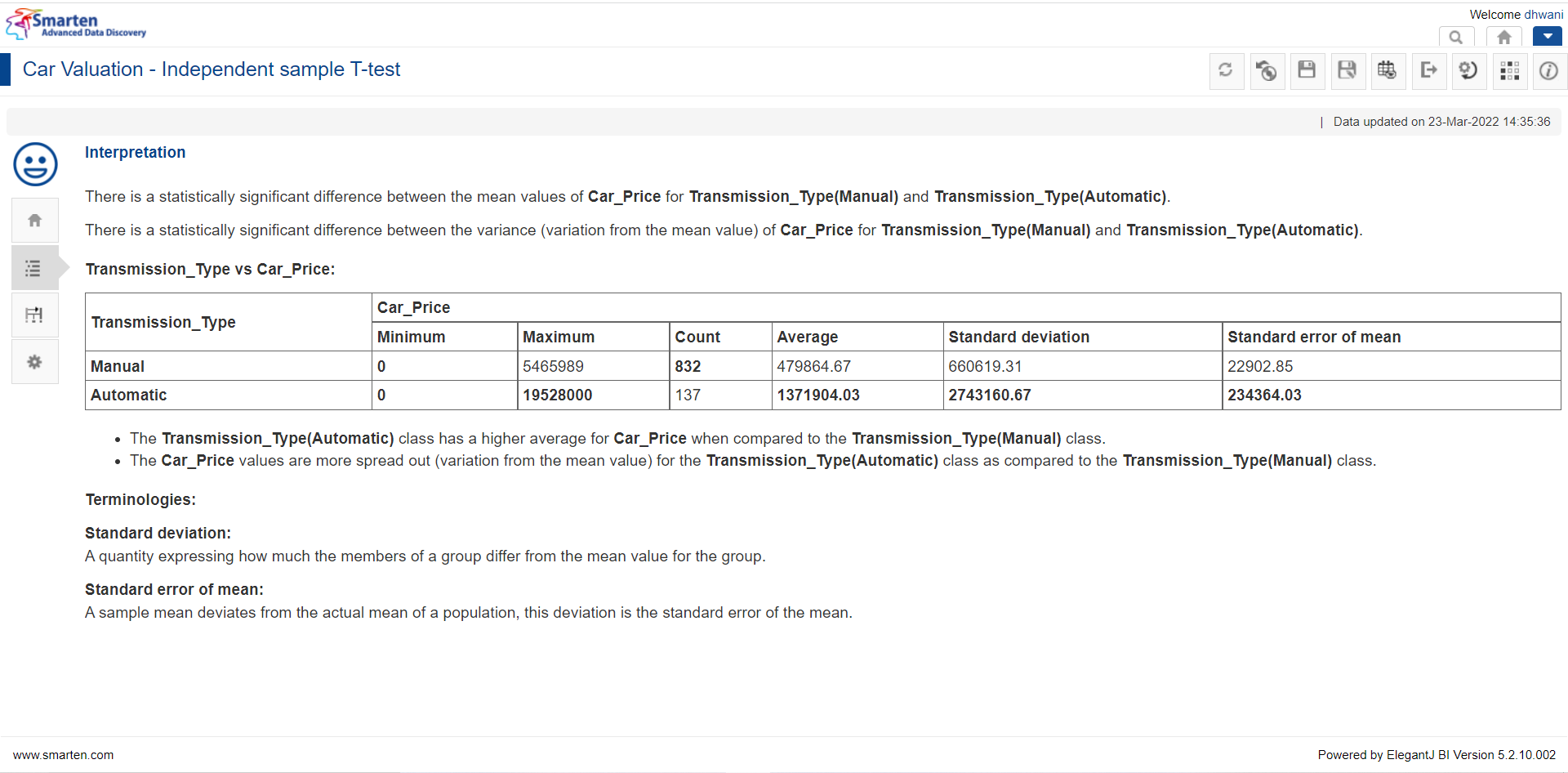Basic Interpretation of Independent samples T-test using Smarten

8.   Model Summary to obtain technical details about Independent samples T-test

In order to obtain a technical understanding about the variation in variables and hypothesis acceptance, the Model Summary tab is provided.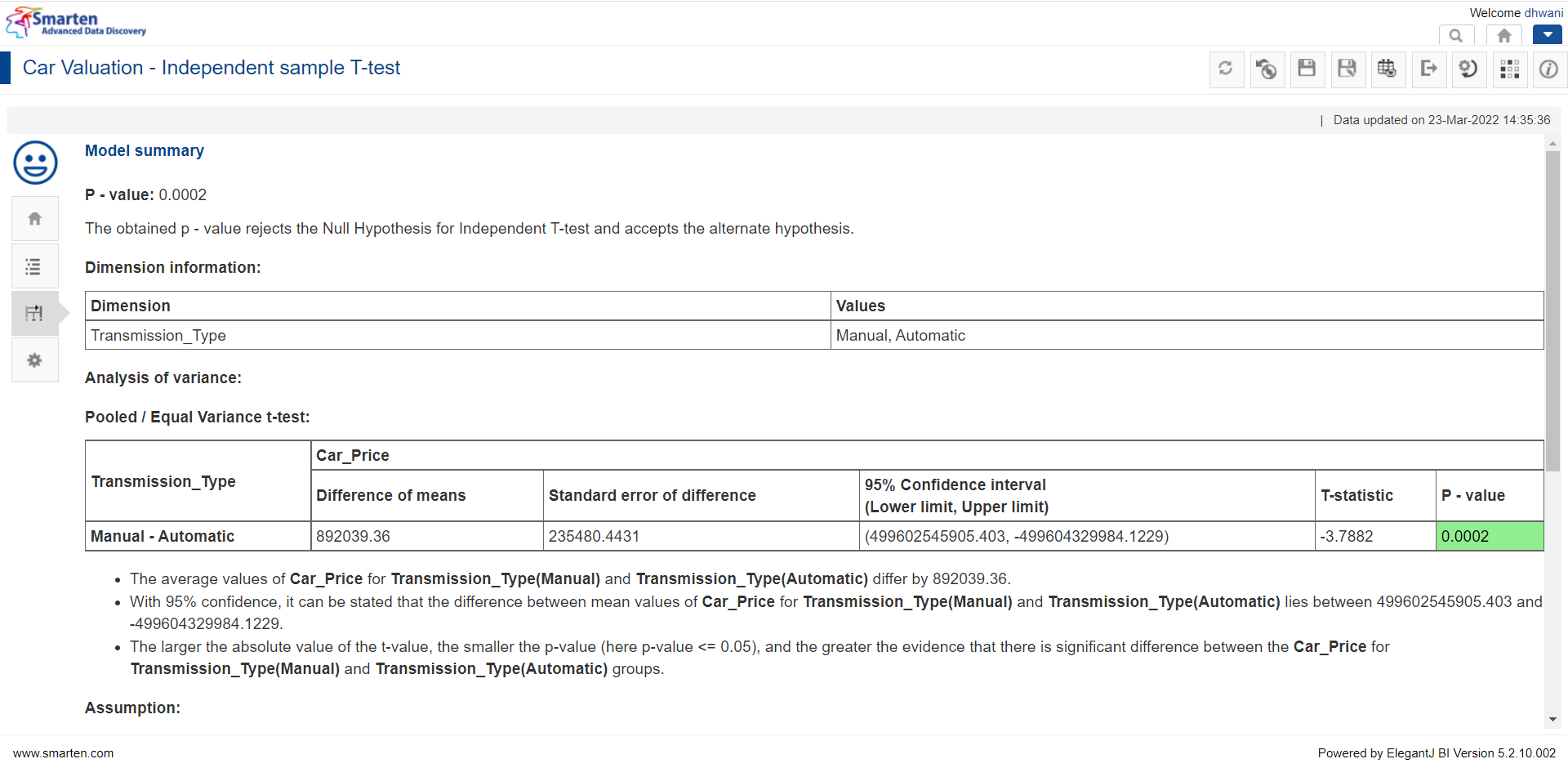Model Summary for Independent samples T-test using Smarten

9.     Finally, yet importantly!

Just as the analysis of car valuation has been made with regards to car transmission type, one can also perform Independent samples T-test on other parameters by changing the model parameters as displayed below: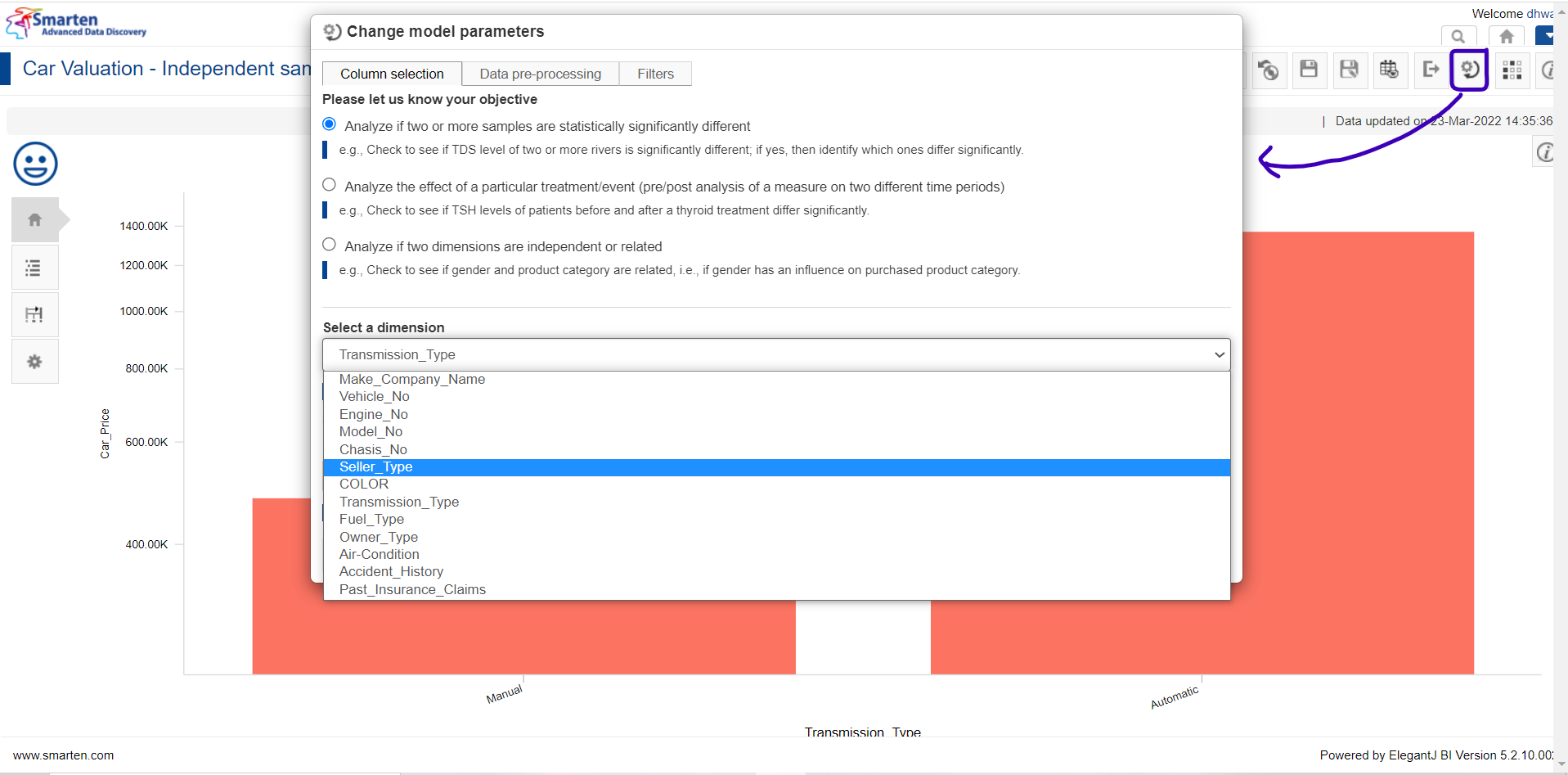Change model parameter using Smarten

Upon choosing a different dimension column, say Seller Type and proceeding, Smarten will automatize the Independent samples T-test and provide us with easy to grasp results from the hypothesis test to obtain noteworthy insights.Independent samples T-test using Smarten

As per the model overview screen, it becomes conclusive that there is no statistically significant difference among the car price based upon the Seller Type of the car, Dealer or an Individual seller have more or less the same selling car prices as per the provided data. Furthermore, the obtained P-value being 0.1003, which is greater than the default significance level of 0.05 for this test, justifiably validates the Null hypothesis (H₀) for Independent samples T-test. It becomes effortless to perform the Independent samples T-test with different parameters (i.e., by changing the model parameters) as well as use-cases (i.e., creating new SmartenInsight for a different use case) to understand the variability among target categorical variable based upon a numeric dependent variable utilizing Smarten Augmented Analytics!